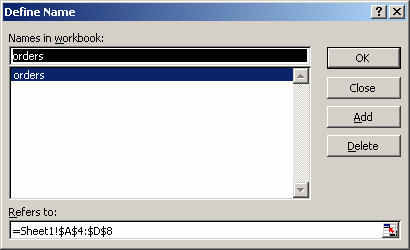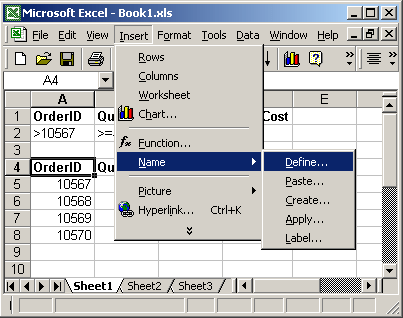# How to Use DMAX Function in ExcelLearn how to use the Excel DMAX function with syntax and examples.

Description

The Microsoft Excel DMAX function returns the largest number in a column in a list or database, based on a given criteria.

## Syntax

The syntax for the Microsoft Excel DMAX function is:

`DMAX( database, field, criteria )`

### Parameters or Arguments

database is the range of cells that you want to apply the criteria against.

field is the column to find the largest number in. You can either specify the numerical position of the column in the list or the column label in double quotation marks.

criteria is the range of cells that contains your criteria.

## Applies To

The DMAX function can be used in the following versions of Microsoft Excel:

• Excel 2013, Excel 2011 for Mac, Excel 2010, Excel 2007, Excel 2003, Excel XP, Excel 2000

## Type of Excel Function

The DMAX function can be used in Microsoft Excel as the following type of function:

• Worksheet function (WS)

## Example (as Worksheet Function)

Let’s look at some Excel DMAX function examples and explore how to use the DMAX function as a worksheet function in Microsoft Excel:Based on the spreadsheet above, the following Excel DMAX examples would return:

 =DMAX(A4:D8, “Unit Cost”, A1:B2) would return \$5.00 =DMAX(A4:D8, 3, A1:B2) would return \$5.00 =DMAX(A4:D8, “Quantity”, A1:A2) would return 12 =DMAX(A4:D8, 2, A1:A2) would return 12

### Using Named Ranges

You can also use a named range in the DMAX function. For example, we’ve created a named range called orders that refers to Sheet1!\$A\$4:\$D\$8.Then we’ve entered the following data in Excel:Based on the spreadsheet above, the following Excel DMAX examples would return:

 =DMAX(orders, “Total Cost”, A1:A2) would return \$35.88 =DMAX(orders, 4, A1:A2) would return \$35.88

To view named ranges: Under the Insert menu, select Name > Define.Credit: http://www.techonthenet.com/excel/formulas/dmax.php